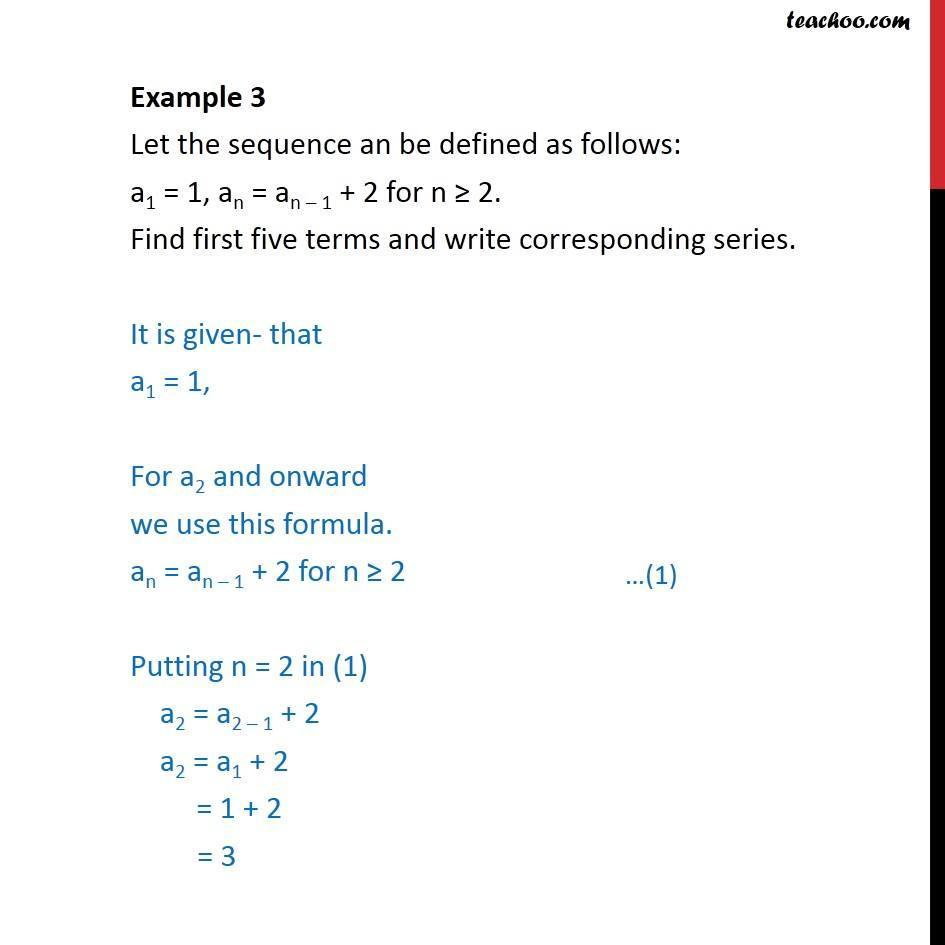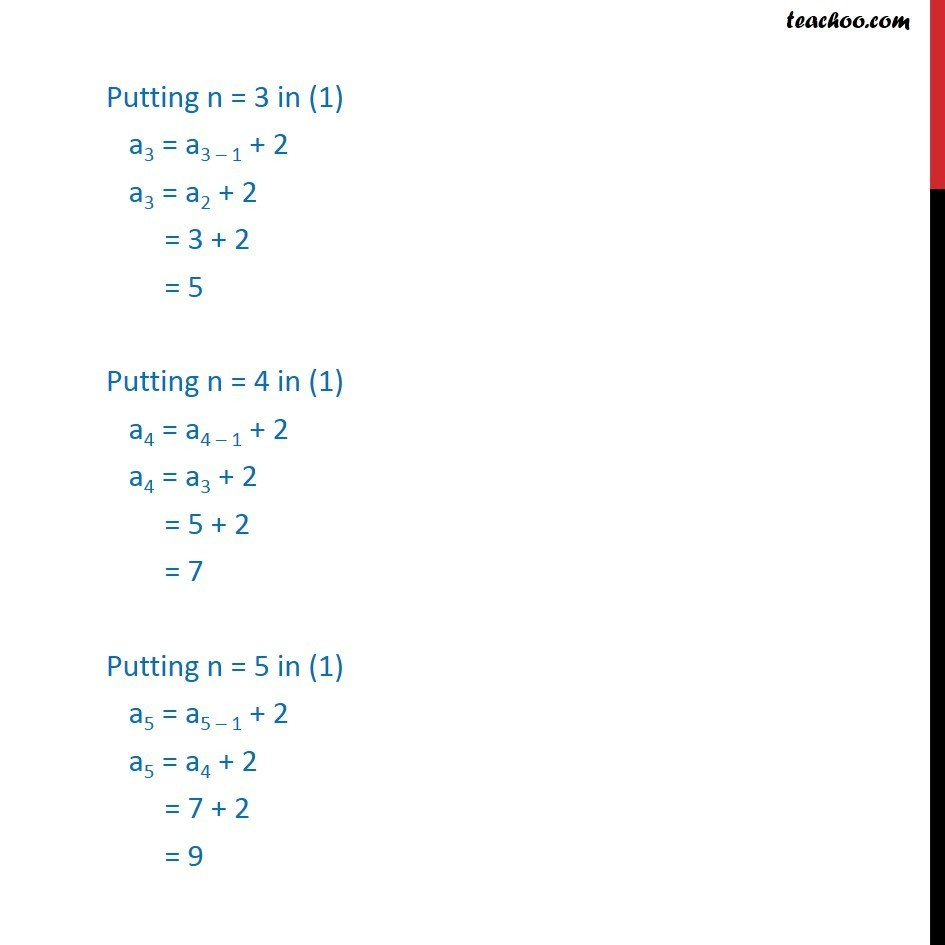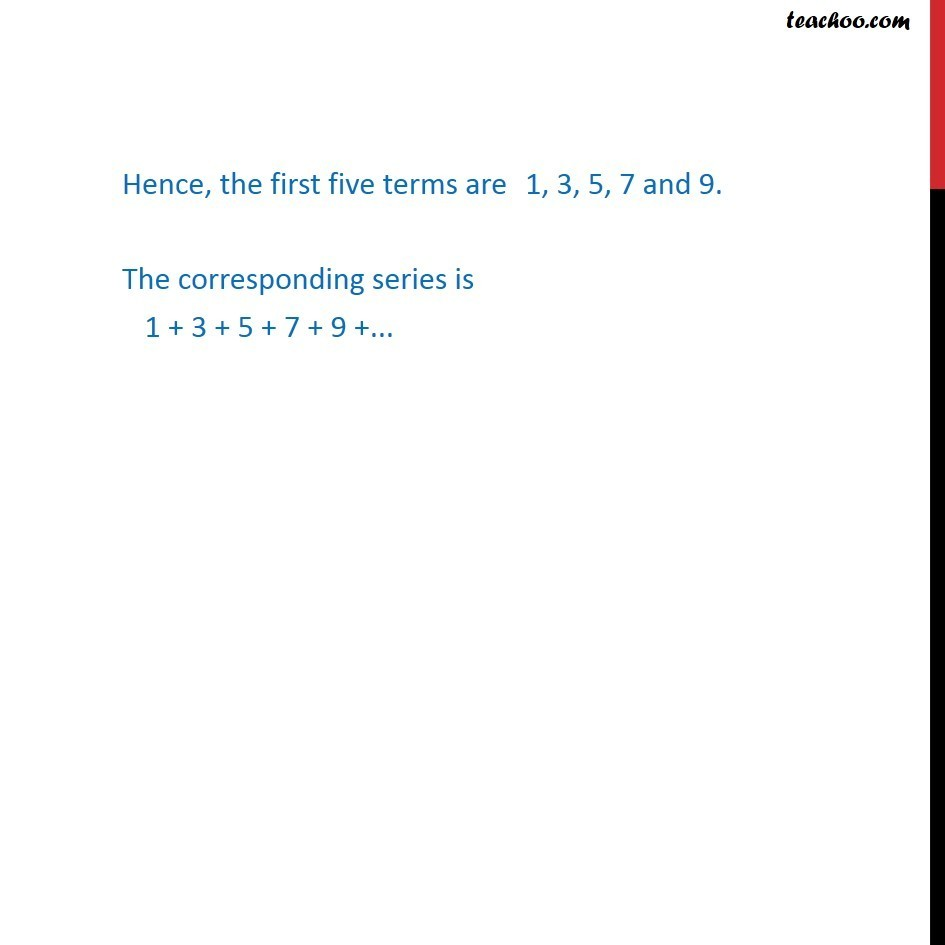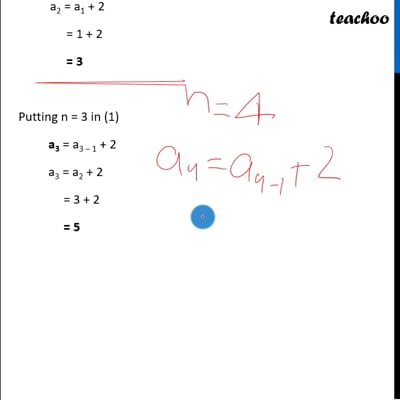Finding sum from series

Chapter 9 Class 11 Sequences and Series
Concept wiseThis video is only available for Teachoo black users

Introducing your new favourite teacher - Teachoo Black, at only ₹83 per month

### Transcript

Example 3 Let the sequence an be defined as follows: a1 = 1, an = an – 1 + 2 for n ≥ 2. Find first five terms and write corresponding series. It is given- that a1 = 1, For a2 and onward we use this formula. an = an – 1 + 2 for n ≥ 2 Putting n = 2 in (1) a2 = a2 – 1 + 2 a2 = a1 + 2 = 1 + 2 = 3 Putting n = 3 in (1) a3 = a3 – 1 + 2 a3 = a2 + 2 = 3 + 2 = 5 Putting n = 4 in (1) a4 = a4 – 1 + 2 a4 = a3 + 2 = 5 + 2 = 7 Putting n = 5 in (1) a5 = a5 – 1 + 2 a5 = a4 + 2 = 7 + 2 = 9 Hence, the first five terms are 1, 3, 5, 7 and 9. The corresponding series is 1 + 3 + 5 + 7 + 9 +...